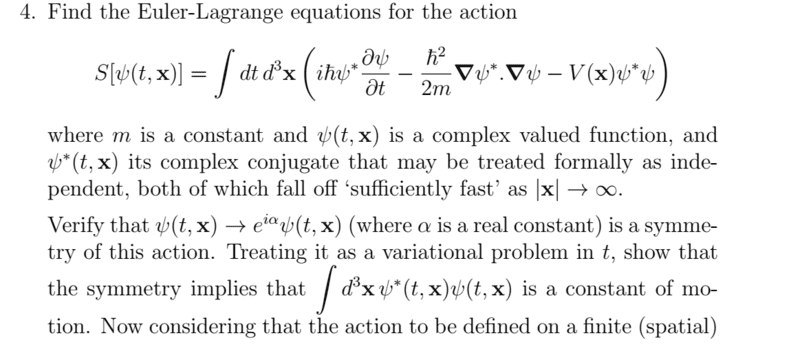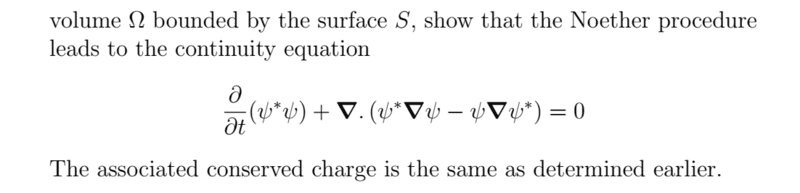# Using symmetry of action to find the constant of motion

Pushoam

## Homework Statement## The Attempt at a Solution

I need help in solving second part of this question.
I put ## e^{i \alpha }\psi ## instead of ##\psi ## and got to see that the integrand doesn't change which means the given transformation is a symmetry of the given action. But how to use this information to find out constant of motion?
I have seen a lot of pdf which talk of the transformation ## \psi ## going to ## \psi + \epsilon \eta (x) ##. Since, the transformation given in this problem is different. I do not know with what I should start.

#### Attachments

•Delta2

I think basically your instructor wants you to show that you understand Noether's proof of her celebrated theorem by applying it in this specific example. Also note that when you carry out the variational procedure (the one usually executed to derive the Euler Lagrange equations of motion) you will get some boundary terms which you need not assume go away. We usually assert $\delta t, \delta x$ are zero on the boundary region. I vaguely recall relaxing this assumption leads to some interesting additional terms having to do with fluxes of conserved quantities across the boundary. But let me emphasize the "vaguely" in that sentence.
•